Write a ratio for the situation in three ways, comparing the first quantity to the second quantity

 Page 1/3 Date 03.09.2017 Size 188.37 Kb. #30093
1   2   3
Level 2 Chapter 5 Study Guide

Multiple Choice

Identify the choice that best completes the statement or answers the question.

Write a ratio for the situation in three ways, comparing the first quantity to the second quantity.

____ 1. An aquarium has 5 whales and 13 sharks.

 a. 13 to 5, 13 : 5, b. 5 to 13, 5 : 13, c. 13 to 18, 13 : 18, d. 5 to 18, 5 : 18,

Express the ratio as a decimal. Round to the nearest hundredth.

____ 2.
 a. 1.49 b. 0.84 c. 1.19 d. 1.01

____ 3. Write in simplest form.

 a. b. c. d.

Write a unit rate for the situation. Round to the nearest hundredth if necessary.

____ 4. driving 140 mi in 2 h 45 min

 a. 0.57 mi/min b. 1.33 mi/min c. 0.85 mi/min d. 0.95 mi/min

____ 5. Population density is the number of people per unit of area. What is the population density of a state that has 1,627,260 people in 1,490 square miles? Round to the nearest whole number.

 a. 10,521 people per square mile c. 1,092 people per square mile b. 1,050 people per square mile d. 109 people per square mile

Use the unit price to find the total price.

____ 6. 28 ft2at \$3.89 per square foot

 a. \$111.44 b. \$71.98 c. \$108.92 d. \$106.40

____ 7. A store sells packages of pencils. Which package offers the best unit price?

 a. 12 pencils for \$3.36 c. 16 pencils for \$4.16 b. 15 pencils for \$4.05 d. 13 pencils for \$3.25

____ 8. Determine which ratio forms a proportion with .

 a. b. c. d.

____ 9. Determine which ratio forms a proportion with .

 a. b. c. d.

____ 10. Determine which ratio forms a proportion with .

 a. b. c. d.

____ 11. Which ratios cannot form a proportion?

 a. , b. , c. , d. ,

____ 12. During one team’s season, the ratio of the number of games the team won to the number of games the team played was 4 to 12. The team played 42 games. How many games did the team win?

 a. 17 games b. 11 games c. 10 games d. 14 games

____ 13. A machine takes 4.2 hours to make 7 parts. At that rate, how many parts can the machine make in 28.8 hours?

 a. 48 parts b. 41 parts c. 172 parts d. 17 parts

____ 14. Solve the proportion.

=

 a. 2 b. 3 c. 1 d. 14

Solve the proportion using cross products. Round to the nearest hundredth if necessary.

____ 15. =

 a. 3 b. 0.003 c. 0.3 d. 30

____ 16.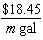=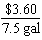a. 38.44 b. 32.54 c. 8.86 d. 42.25

____ 17. Are the figures below necessarily similar? Why or why not?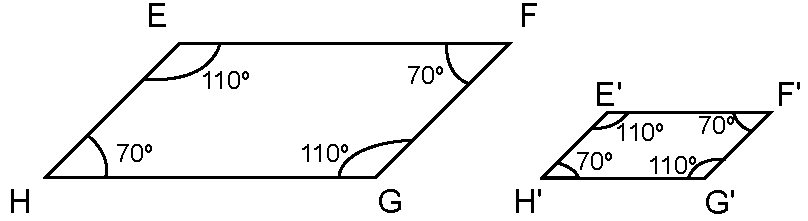a. No, because the corresponding angles are not necessarily congruent and the corresponding sides are not necessarily proportional. b. Yes, because the corresponding angles are congruent and the corresponding sides are proportional. c. No, because the corresponding angles are not necessarily congruent. d. No, because the corresponding sides are not necessarily proportional.

____ 18. Are the figures below similar? Why or why not?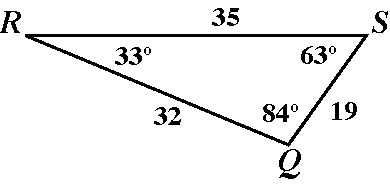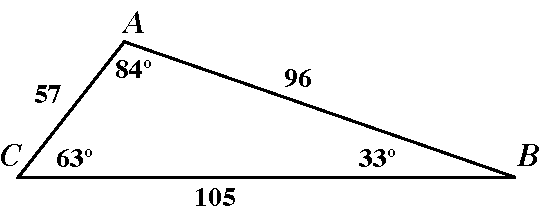a. Yes, because the corresponding angles are congruent and the corresponding sides are proportional. b. No, because the corresponding sides are not proportional. c. No, because the corresponding angles are not congruent. d. No, because the corresponding angles are not congruent and the corresponding sides are not proportional.